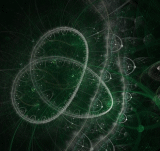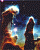xString theory
My hypothesis on gravity, 5d space time and hallucinations
Posts  1 - 1  of  1anujthehacker
After giving a lot of thought, i'll finally post my hypothesis (research) that i have been working on since approx 2 years online. Which is about Gravity, 5 Dimensions of space - time and Time travelling. I want every one of you to read it patiently and have a view of my understanding of this universe.

Happy Reading Friend

Hypothesis on Gravity and 5D space-time

Whatever we see around is matter. We see matter because we live in a 4 dimensional universe according to present day science. That means 3 dimensions of space and 1 dimension of time. Everything is materialised and controlled by positive direction in time.

What if we could go back and forth in time? That pretty much means controlling time.

As we can choose to have N number of dimensions in a hypothesis, we will assume 5 dimensions, i.e. 3 spatial dimensions and 2 dimensions of time. We will also consider our brains to be the most complex entity in the entire universe, which can have access to a 5D universe by dreaming and sometimes hallucinating ( projections of our consciousness in 5D plane, into our visions and ears ) by sleeplessness, psychedelic drugs or schizophrenia(in medical science, it is a disease which has hallucinations as its major symptom). Our dreams are the plane with infinite time that we experience through quantum entanglement. Our consciousness by is allowed to move around in this 5 dimensional structure made by packets of universes and connected by a constant of gravity. Yes, we'll assume gravity to be a constant throughout.

Lets talk about our own universe. How did it start? I'll refer to super string theory for further explanations. Well, according to super string theory, our universe is made up of strings. All matter is made up of infinitely small 2 dimensional strings which vibrate to form baryonic matter.

Our universe on the other hand, is also the result of an expansion of one of the strings. Which created a brane (membrane, which is an expansion of a string) filled with space, positive direction in time. This expansion is what we know as The Big Bang. There was just energy in the beginning. A bright place filled with nothing but just photons. Photons then created equal amount of matter and antimatter, hence, forming our known universe.

What about if we dream and we remember a dream and use the information from the 5D plane which has infinite space and time to change something in this plane. Our consciousness is getting connected to the 2D plane by means of gravity. Our consciousness then transition to the 5D plane. Now it has access to infinite time and the entire space, as it has access to the whole connection of strings (parallel universes). Our consciousness gets mature by experiencing 2 dimensions of time as it then gets altered from the manifestation and receives information from the subset of the 5 dimensional world which is 2 dimensional time If we can change the orientation of our dreams to the one which obeys our laws of physics, then we can increase the maturity of our consciousness to project the state of the one that has travelled in time in maybe the positive or the negative direction.

The process of maturity of consciousness happens through quantum entanglement of the consciousness with the string through gravity (fabric of space). Which allows teleportation through gravity waves at the speed of light by swapping quantum information of 2 places at different location in time axis.

The fabric of space time is not curved, it is actually stretched. Strings expand into branes which stretch space creating 3D space-time. Every string is an opening to a 5D space-time which is in fact a possibility of our universe. Each string is a packet of this 5D universe.

When a string expands to form a brane, it is disorienting the time to move in one direction, which restricts it to not to be able to move throughout the 2D plane of time. The expansion allows the Higgs field to interact with the expanded brane and thus form mass proportional to the expansion of the string.

All the energy, is the amount of all the strings present in this universe. Light (photon) is the quanta of this universe. Each packet of light is a possibility. Energy gets converted into matter due to expansion of string, and expansion of string further stretches space. So a string moves in positive x-axis of time when expanded into a brane.

All the strings in our universe is connected to each other, creating a network of 5D plane and each string contains opening to a 2D plane with infinite time. Gravity is the wire which forms this network of strings.

We live in a 5D universe but are allowed to either experience 4 of them or 5 space time dimensions that a string induces. So we live parallel to a plane that has 3 dimensions of space and 2 dimensions of time.

When we dream, our consciousness is connected to the plane of 5D space-time and hence, we can have access to infinite space-time. Our dreams are the way they are, because of improper orientation of dreams. They are not bounded by the laws of physics we are bounded by. Hence, they are so weird in some sense.

Einstein in his special theory of relativity said that when we move close to the speed of light the flow of time decreases by the formula -

Delta t' = Delta t/(1-v^2/c^2)

Time dilation calculated by the formula is correct in every aspect. But when we talk about time moving in 2 dimensional plane
We'll use the formula -

We'll use derivation

delta T0 = deltaT/(1-v^2/c^2)^-1/2 (Time Dilation, by Albert Einstein)

(1-v^2/c^2)^-1/2 = deltaT/deltaT0

1-v^2/c^2=(DeltaT/deltaT0)^2

v^2/c^2=1-(DeltaT/deltaT0)^2

v^2/1-(DeltaT/deltaT0)^2 = c^2 —(1)

E = m c^2 (Theory of Special Relativity by Albert Einstein)

m = E/c^2 —(2)

E = (1/2)mv^2 —(3)

substituting (2) in (3)

E = Ev^2/c^2 —(4)

now substituting (1) in (4)

E = E2(v^2/v^2)1-(DeltaT/deltaT0)^2

+ and - of 1/2^1/2 = DeltaT/DeltaT0

Delta T = + and - of 2^-1/2 Delta T0

Delta T0 = + and - of 2^1/2 Delta T

delta T0 subscript(x,y) = + and - of 2^1/2 deltaT subscript(x,y)

where x,y are 2D co-ordinates of delta T

We move perpendicular to the time axis when we move with speed close to light, which means that we move in imaginary time.

When photons enter our retina, they form an image of the 3D plane but when in 5D plane, we receive quantum information of the particle in infinite time. Every string has a quantum information for each string in this web of fabric of 5D space-time.

Strings in fact are the openings of of a 2D plane that shares dimension of this plane and the 2D plane of time. Branes are in fact the expansion of a string which conceives particles, which are the stretches in space that form visible matter.

Photons is the exception of the theory. Photons always travel at the speed of light.
They have characteristics of both a 3D particle and a 5D wave. A photon has relative mass so it interacts with gravity. But because of no rest mass it is not bounded by time. It has access to infinite amount of it. It has access to infinite time so, it is a particle that is not bigger than a value which is sufficient enough for it to interact with the Higgs field (Higgs field is a field proposed by the scientist Peter Higgs, which is a field which lends mass to all the particles of the standard model of physics), so photons with no rest mass, doesn’t exert the force of gravity to other objects.

As a photon has access to infinite amount of time, it gets converted into matter and anti-matter particle pairs (bi-polar couples) when energy(string) expand to conceive a brane(matter) with matter particle moving in positive direction of time and the anti particle of it moving in the negative direction according to Richard Feynman.

All the energy that is either in this universe or is some how getting converted into energy by matter by the formula E=MC^2 behaves like a photon. When energy gets converted into matter(3 dimensional brane), it is restricted to just 1 direction in time, which is the positive direction.

In the beginning I introduced a constant. The constant is gravity which is actually the curved space we have our reality founded on. It is weak in our universe (brane) because matter just experience positive direction of time. So it experiences just 1/infinite part of the real strength of gravity.

A black hole is a space-time singularity with infinite amount of density and proportional gravitational field which is infinite too. Which is a hole in space time which is caused when an object with that amount of density becomes as big as the swarzchild radius. And the flow of time freezes which in turn means infinite time getting leaked in our universe which in turn means information from the 2 Dimensional plane getting leaked which results in ripples in space-time called gravity waves which carry information away from a merged black hole that is formed when a pair of blackhole collides, creating ripples in space time proposed by Albert Einstein in his theory of General Relativity.

Pineal Gland is a gland in our brains which secretes a substance called serotonin, which is photosensitive and gets its stimulus from darkness. Serotonin also regulates our dream patterns. So, this is how we experience 5D plane. The gland also sometimes secretes DMT ( di-methyl triptamine ). Which causes visual and auditory hallucinations, which means experiencing 3 spatial and 2 time dimensions. Hallucinations are created by a person's own consciousness, so, only the one who experiences 5D will see them.

According to Quantum Superposition principle, there are infinite ways in which a system can occur or there are infinite probable ways in which a system can react.
Infinite possibilities are the infinite amount of space-time we experience in this universe.

Hallucinations happens when our consciousness is connected to infinite space and time. We are connected to all the matter in this universe in present as well as past and future. The baryonic matter we are familiar of is the expanded string (brane) that hold within infinite space-time. When we are accessing infinite space-time, we are quantum entangled with other branes and have access to the frequencies of the vibration of branes. Each brane has a specific frequency in which it vibrates.

The next level of schizophrenia is projecting hallucinations into our universe by quantum entanglement. Which is projecting of auditory hallucinations and even getting them record in devices. And the subsequent level would be controlling matter and then even time and it will go on until our body gets completely converted into consciousness.

This is evolution according to Darwin's theory of evolution. We evolve when information is passed on by DNA when we choose to reproduce when the environment is favourable. Maybe schizophrenia is the next level of evolution of homosapiens since the first female australopithecus (lucy). Amusing and interesting.

Nothing is actually the way it is. Everything is mere perception which one has.